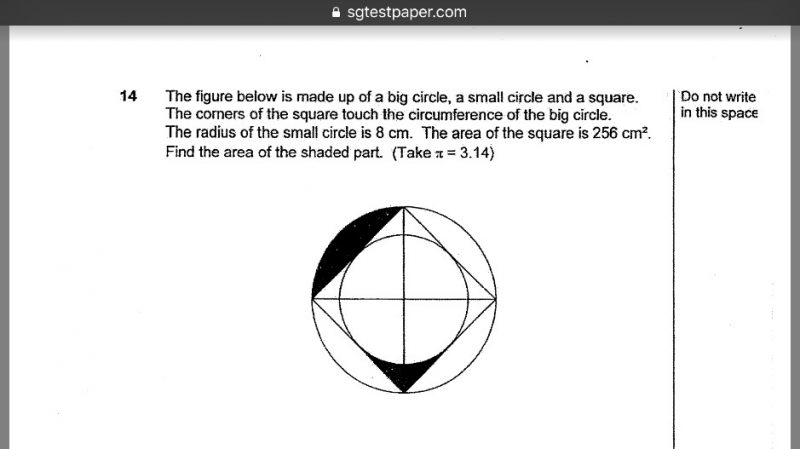# QuestionNeed help with detailed working. Can’t u/S some of the given solns

mgs

1 Answer

# Answer

The area of ¼ of the square = 256 ÷ 4 = 64 cm²

Let R cm be the radius of the larger circle.

Since ¼ of the square is a right-angled triangle with base and height of R cm, the area of the triangle = ½ × R × R = ½R² cm²

So ½R² = 64

R² = 128

So the area of the larger circle = πR² = 128π

The area of the shaded part in the top left hand side of the figure = (Area of the larger circle – Area of the square) ÷ 4 = (128π – 256) ÷ 4 = (32π – 64) cm²

The area of the shaded part at the bottom = (Area of square – Area of the smaller circle) ÷ 4 = (256 – π8²) ÷ 4 = (64 – 16π) cm²

Total area of the shaded parts = (32π – 64) + (64 – 16π) = 16π = 16 × 3.14 = 50.24 cm²

0 Replies 0 Likes